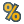You are viewing the RapidMiner Studio documentation for version 8.1 - Check here for latest version

#T-Test (RapidMiner Studio Core)

## Synopsis

This operator is used for comparison of performance vectors. This operator performs a t-test to determine the probability for the null hypothesis i.e. 'the actual means are the same'.

## Description

The T-Test operator determines if the null hypothesis (i.e. all actual mean values are the same) holds for the given performance vectors. This operator uses a simple paired t-test to determine the probability that the null hypothesis is wrong. Since a t-test can only be applied on two performance vectors this test will be applied to all possible pairs. The result is a significance matrix.

Paired t-test is a test of the null hypothesis that the difference between two responses measured on the same statistical unit has a mean value of zero. For example, suppose we measure the size of a cancer patient's tumor before and after a treatment. If the treatment is effective, we expect the tumor size for many of the patients to be smaller following the treatment. This is often referred to as the 'paired' or 'repeated measures' t-test.

In case of this operator the dependent samples (or 'paired') t-tests consist of a pair of performance vectors. Doing multiple paired t-tests would result in an increased chance of committing a type I error. 'False positive' or Type I error is defined as the probability that a decision to reject the null hypothesis will be made when it is in fact true and should not have been rejected. It is recommended to apply an additional ANOVA test to determine if the null hypothesis is wrong at all. Please use the ANOVA operator for performing the ANOVA test.

## Differentiation

###ANOVA

Doing multiple two-sample t-tests would result in an increased chance of committing a type I error. For this reason, ANOVA is useful in comparing two, three, or more means.

## Input

•performance (Performance Vector)

This operator expects performance vectors as input and can have multiple inputs. When one input is connected, another performance input port becomes available which is ready to accept another input (if any). The order of inputs remains the same. The performance vector supplied at the first input port of this operator is available at the first performance output port of the operator.

## Output

•significance (T-Test Significance)

The given performance vectors are compared and the result of the significance test is delivered through this port.

•performance (Performance Vector)

This operator can have multiple performance output ports. When one output is connected, another performance output port becomes available which is ready to deliver another output (if any). The order of outputs remains the same. The performance vector delivered at the first performance input port of this operator is delivered at the first performance output port of the operator.

## Parameters

• alphaThis parameter specifies the probability threshold which determines if differences are considered as significant. If a test of significance gives a p-value lower than the significance level alpha, the null hypothesis is rejected. It is important to understand that the null hypothesis can never be proven. A set of data can only reject a null hypothesis or fail to reject it. For example, if comparison of two groups reveals no statistically significant difference between the two, it does not mean that there is no difference in reality. It only means that there is not enough evidence to reject the null hypothesis (in other words, the experiment fails to reject the null hypothesis). Range: real

## Tutorial Processes

### Comparison of performance vectors using statistical significance tests

Many RapidMiner operators can be used to estimate the performance of a learner or a preprocessing step etc. The result of these validation operators is a performance vector which collects the values of a set of performance criteria. For each criterion, the mean value and standard deviation are given. The question is how can these performance vectors be compared? Statistical significance tests like ANOVA or T-Test can be used to calculate the probability that the actual mean values are different. This Example Process performs exactly the same task.

This Example Process starts with a Subprocess operator which provides two performance vectors as output. Have a look at the inner operators of the Subprocess operator. The Generate Data operator is used for generating an ExampleSet. The Multiply operator is used for producing multiple copies of this ExampleSet. X-Validation operators are applied on both copies of the ExampleSet. The first X-Validation operator uses the Support Vector Machine (LibSVM) operator whereas the second X-Validation operator uses the Linear Regression operator in the training subprocess. The resultant performance vectors are the output of the Subprocess operator.

These performance vectors are compared using the T-Test and ANOVA operators respectively. The performance vectors and the results of the significance tests are connected to the result ports of the process and they can be viewed in the Results Workspace. Run the process and compare the results. The probabilities for a significant difference are equal since only two performance vectors were created. In this case the SVM is probably better suited for the data set at hand since the actual mean values are probably different. SVM is considered better because its p-value is smaller than alpha which indicates a probably significant difference between the actual mean values.